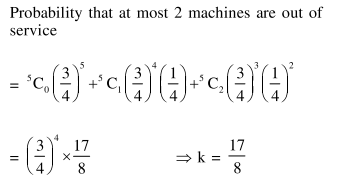# In a workshop,

Question:

In a workshop, there are five machines and the probability of any one of them to be out of

service on a day is $\frac{1}{4}$. If the probability that at most two machines will be out of service on the same day is $\left(\frac{3}{4}\right)^{3} \mathrm{k}$, then $\mathrm{k}$ is equal to :

1. $\frac{17}{2}$

2. 4

3. $\frac{17}{8}$

4. $\frac{17}{4}$

Correct Option: , 3

Solution: